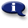# .

## Syllabus Information

Winter 2022
Aug 12,2022Use this page to maintain syllabus information, learning objectives, required materials, and technical requirements for the course.

Syllabus Information
MTH 052 - Math for Health and Physical Sciences
Associated Term: Winter 2022
Learning Objectives: Upon successful completion of this course, the student should be able to: 1. Practice decimal and fraction operations and apply to solve applications. 2. Demonstrate the correct use of English, metric and apothecary units of measurement. 3. Solve problems using unit (dimensional) analysis. 4. Apply concepts of approximate numbers for rounding measurements. 5. Demonstrate the correct use of labels for measurements, applications, and graphs. 6. Use basic geometric formulas for area and perimeter of quadrilaterals. 7. Compute with signed numbers. 8. Solve equations and formulas for one variable. 9. Interpret and create line graphs. 10. Calculate and label slope of line graphs. 11. Solve problems involving percents. 12. Solve problems involving proportions for solutions and variation. 13. Use and compare Fahrenheit, Celsius, and Kelvin temperature scales. 14. Find the volume and density of objects. 15. Calculate pH quantities involving logarithms. 16. Calculate dosages for oral meds and injected meds including reconstituted liquids. 17. Use and compute with exponential and scientific notation. 18. Calculate both by hand and scientific calculator when appropriate.
Required Materials:
Technical Requirements: